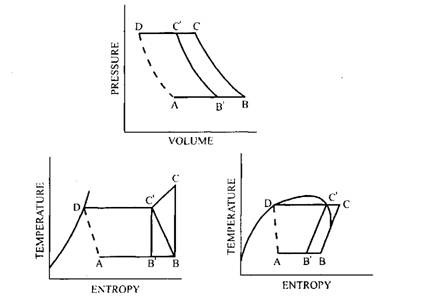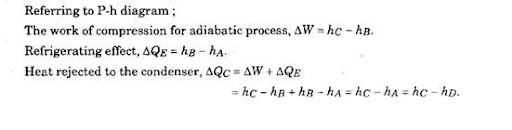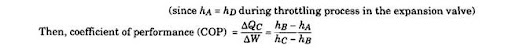# Theoretical Vapour Compression Cycle (Automobile)

33.4.

## Theoretical Vapour Compression Cycle

The theoretical vapour compression cycle is the reverse of the Rankine cycle applied for power plants. Figure 33.4 shows the theoretical cycle on pressure-volume (P-V), temperature-entropy (T-s) and pressure-enthalpy (P-h) coordinates. The cycle works between the tempera­tures, Ti and Ti and pressures Pi and P2 representing the condenser and evaporator states respectively.Fig. 33.4. Vapour compression refrigeration cycle.
The various processes of the cycle A-B-C-D are as presented below :

(i) Process B-C. Isentropic compression of the vapour takes place from state B to C. If vapour state is saturated (J3) or superheated, the compression is called dry compression. If initial state is wet (B’) the compression is called wet compression, as represented by B’ – C.
Hi) Process C-D. Heat rejection in condenser is at constant pressure. It is carried out in two stages. The first is through C-C where heat is rejected by desuperheating of the vapour and the second is C – D where latent heat is rejected at constant temperature.
(Hi) Process D-A. An irreversible adiabatic expansion of vapour takes place through the expansion valve. The pressure and temperature of the liquid are reduced. The process is accompanied by partial evaporation of some liquid.
(iv) Process A-B. Heat absorption in the evaporator takes place at constant pressure. The final state depends on the quantity of heat absorbed and the same may be wet (B’), dry (B) or superheated.